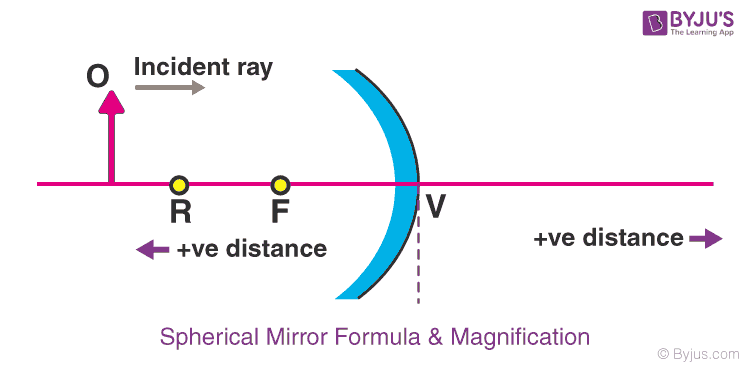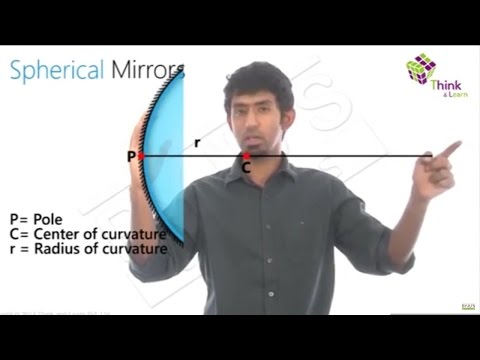Checkout JEE MAINS 2022 Question Paper Analysis : Checkout JEE MAINS 2022 Question Paper Analysis :

# Spherical Mirror Formula & Magnification

Spherical mirrors are the mirrors having curved surfaces that are painted on one of their sides. Spherical mirrors in which inward surfaces are painted are known as convex mirrors while the mirrors in which outward surfaces are painted are known as concave mirrors.Concave mirrors are also known as converging mirrors since the rays converge after falling on the concave mirror while, the convex mirrors are known as diverging mirrors as the rays diverge after falling on the convex mirror.

Let’s learn about formula and magnification produced by spherical mirrors.

## Spherical Mirror FormulaThe Mirror formula explains how object distance (u) and image distance (v) are related to the focal length of a spherical mirror. Object distance is the distance of the object from the pole of the mirror; denoted by the letter u. Image distance is the distance of the image from the pole of the mirror and it is denoted by the letter v. And focal length is the distance of the principal focus from the pole of the mirror. The expression which gives the relation between these three quantities is called the mirror formula which is given as:

$$\begin{array}{l}\frac{1}{v}+\frac{1}{u}=\frac{1}{f}\end{array}$$

Mirror formula is applicable for all spherical mirrors for every position of the object.

## Magnification by Spherical Mirrors

Magnification is the increase in the image size produced by spherical mirrors with respect to the object size. It is the ratio of the height of the image to the height of the object and is denoted as m. The magnification, m produced by a spherical mirror can be expressed as:

$$\begin{array}{l}m=\frac{h}{{h}’}\end{array}$$

Here, h is the height of image and h’ is the height of the object.

Magnification is also equal to the ratio of image distance to the object distance.

$$\begin{array}{l}m=\frac{v}{u}\end{array}$$

As the object is always above the principal axis, the height of the object is always positive. But a sign for image height may vary according to the type of image formed. The height of virtual images should be taken positive while the height of real images should be taken negatively.

## Uses of Magnification

• A precision magnifier serves the role of a simple magnifier but holds multiple elements to erase aberrations and yield a sharper image.
• A water droplet acts as a simple magnifier that magnifies the object behind it. The water forms spherical droplets due to the influence of surface tension. When the droplet is in contact with an object, a spherical shape is distorted but capable of forming an image.

Points to remember:

• The positive magnitude of magnification indicates virtual and erect image.
• The negative magnitude of magnification indicates real and inverted image.## Frequently Asked Questions – FAQs

### What is a spherical mirror?

A spherical mirror is a mirror that has the shape of a piece cut out of a spherical surface.

### What are the two types of spherical mirrors?

The two main types of spherical mirrors are convex and concave mirrors.

### What is meant by the centre of curvature?

The point in the centre of the mirror passes through the curve of the mirror and has the same tangent and curvature at that point.

### Define the principal axis of a mirror.

It is the imaginary line that passes through the optical centre and the centre of curvature of any lens or a spherical mirror.

### What are the uses of magnification?

A precision magnifier functions the role of a simple magnifier but holds multiple elements to erase aberrations and result in a sharper image.
A water droplet functions as a simple magnifier that magnifies the object behind it. The water forms spherical droplets due to the influence of surface tension. When the droplet is in contact with an object, a spherical shape is distorted but capable of forming an image.

Test Your Knowledge On Spherical Mirror Formula!

#### 1 Comment

1. This is a very helpful site for students (and even teachers)